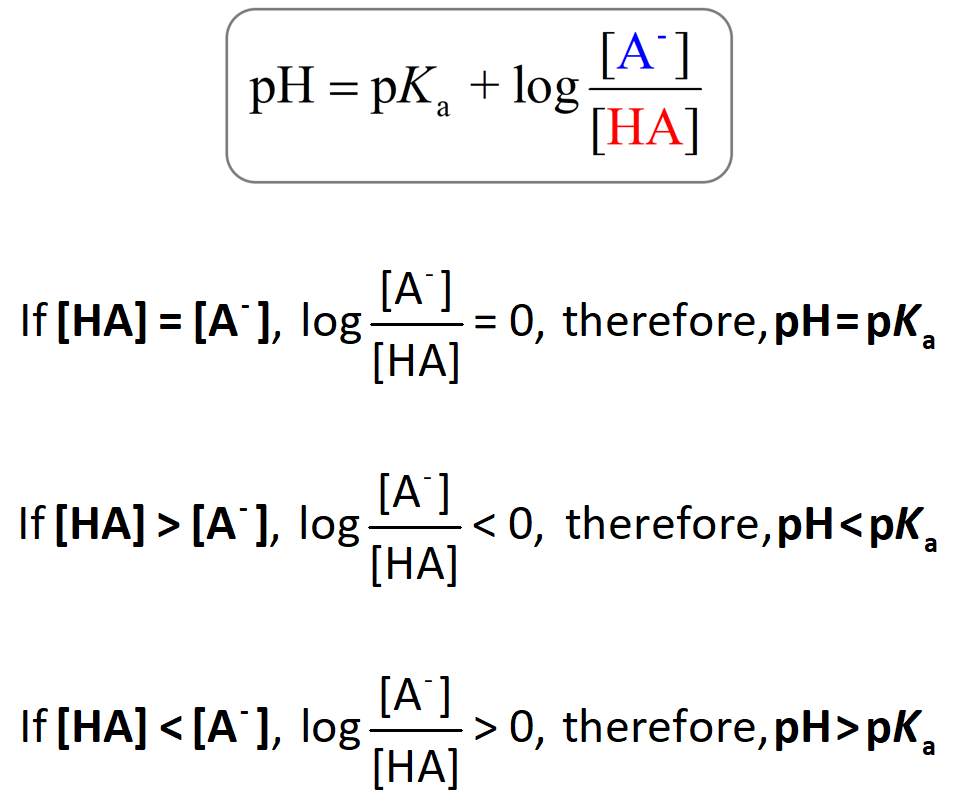## General Chemistry

The Henderson–Hasselbalch equation shows the correlation of the pKa and pH of the buffer solution.

The pKa of a given acid, or the conjugate acid of the base, is constant and therefore, the only variable in the equation is the logarithm term which depends on the acid-base pair concentrations.

One application of this relationship is the possibility to predict whether the pH of the solution is going to be equal to or different than the pKa of the acid. There are three scenarios here:

1) when [HA] = [A], 2) [HA] > [A], and 3) [HA] < [A].

1) When [HA] = [A], the logarithm becomes zero, and therefore, the pH = pKa.

2) [HA] > [A]: If there is more acid than its conjugate base in the solution, then the pH will be smaller than the pKa (pH < pKa) because the logarithm is a negative number. This also makes sense intuitively; more HA means more acid, and thus a lower pH.

3) [HA] < [A]: If the conjugate base is present in a larger quantity, the solution goes more basic because the logarithm now is greater than zero. So, in this case, pH > pKa:For example, calculate the pH of a solution consisting of 0.65 M NH4Cl and 0.25 M NH3. Kb (NH3) = 1.8 x 10-5.

So, right from the concentrations of the base (NH3), and its conjugate acid, we can see that [HA] > [A], therefore the pH is going to be smaller than the pKa. Let’s confirm this by doing the calculations too.

If the pKa of the acid is not given, you need to determine it from wither the Ka/Kb, or  pKa/ pKb relationship. Let’s use the pKa/ pKb relationship this time:

pK= -log Kb = -log 1.8 x 10-5 = 4.75

pKa + pKb = 14

pKa = 14 – pK= 14 – 4.75 = 9.25

And now that we know the pKa and the concentrations of HA and A, we can calculate the pH using the Henderson–Hasselbalch equation:

${\rm{pH}}\; = \;{\rm{p}}{K_{\rm{a}}}\;{\rm{ + }}\;{\rm{log}}\frac{{{\rm{[N}}{{\rm{H}}_3}{\rm{]}}}}{{{\rm{[N}}{{\rm{H}}_4}{\rm{Cl]}}}}\;$

${\rm{pH}}\; = \;9.25\; + \,{\rm{log}}\frac{{{\rm{0}}{\rm{.25}}\;M}}{{{\rm{0}}{\rm{.65}}\;{\rm{M}}}}\; = \;8.84$

As expected, pH < pKa (8.84 vs 9.25) because [HA] > [A].

## An example when the concentration of the acid is smaller than the conjugate bases:

Calculate the pH of a solution containing 0.25 M HF and 0.55 M NaF. pKa (HF) = 3.8.

This time we have the pKa, so all we need to do is plug in the numbers:

${\rm{pH}}\; = \;{\rm{p}}{K_{\rm{a}}}\;{\rm{ + }}\;{\rm{log}}\frac{{{\rm{[}}{{\rm{F}}^{\rm{ – }}}{\rm{]}}}}{{{\rm{[HF]}}}}$

${\rm{pH}}\; = \;{\rm{3}}{\rm{.8}}\;{\rm{ + }}\,{\rm{log}}\frac{{{\rm{0}}{\rm{.55}}\;{\rm{M}}}}{{{\rm{0}}{\rm{.25}}\;{\rm{M}}}}\;{\rm{ = }}\;{\rm{4}}{\rm{.14}}$

And this confirms that if [HA] < [A] then pH > pKa.

Check Also